Like   Tweet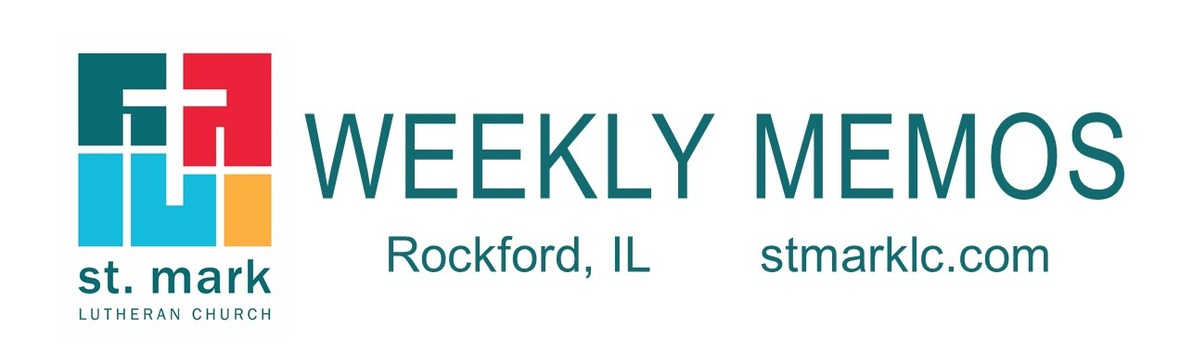table div table+table div table{width:100%;padding:0}table div table+table div table img{width:96.23%;padding:0;float:none}table div table+table div table td{width:100%;padding:0 1.88% 18px}/* styles */## News and Events

 table div table+table+table+table div table{width:100%;padding:0}table div table+table+table+table div table img{width:96.23%;padding:0;float:none}table div table+table+table+table div table td{width:100%;padding:0 1.88% 18px}/* styles *//* styles */ Drive-In Outdoor Worship Service Sunday, October 18 Come join us this Sunday for the 20th Sunday after Pentecost at 9:30 A.M. at St. Mark in the south parking lot. If you are away or unable to go out in public, the service will be live-streamed on our St. Mark YouTube Channel. The link will be emailed to you Sunday morning at 8:00 A.M. and then available for you to watch again and again later in the day, just like our other worship videos. We should mention that for safety reasons our Mulford Road entrance will be closed during these services. When you arrive, you will want to enter via the Featherstone Road entrance. There will be volunteers handing out bulletins and communion wafers. Ushers will guide you to where you need to go. At the end, you can leave either the same way you entered or through the adjoining OSF parking lot along with a bag of donut holes. We can't wait to see you on Sunday, October 18!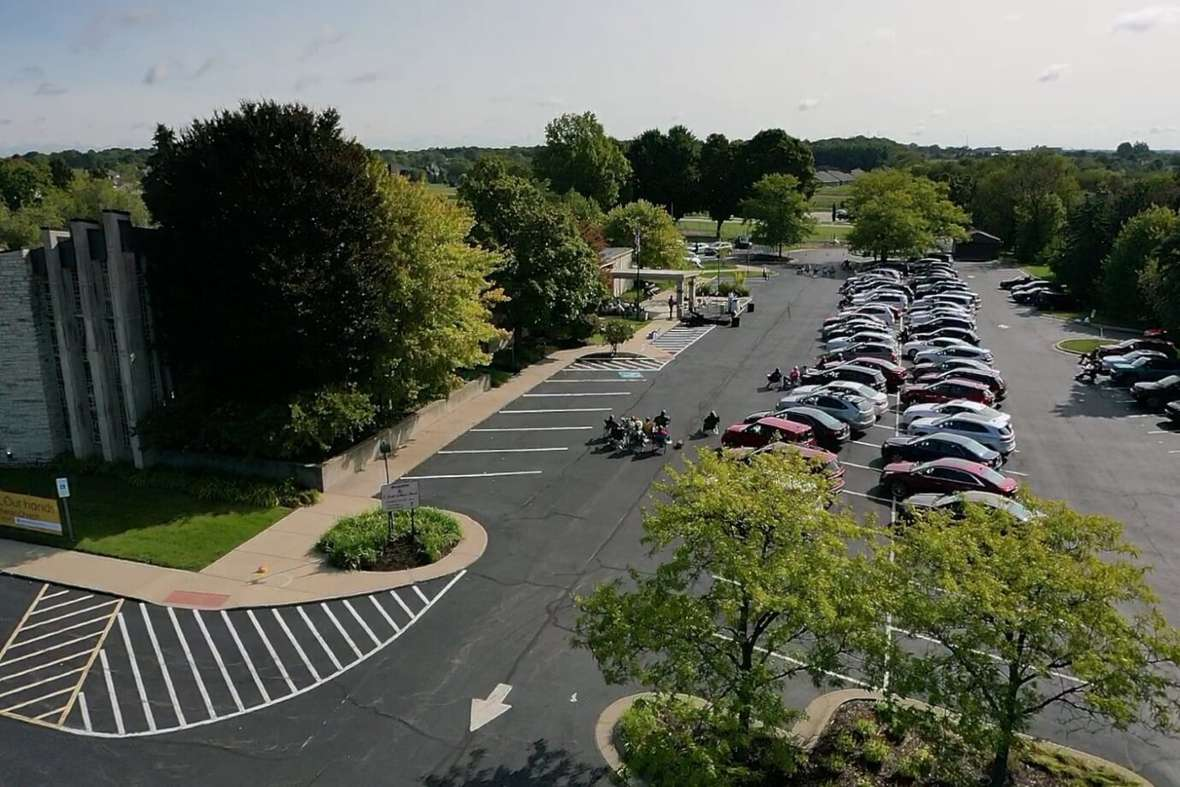table div table+table+table+table+table+table+table div table{width:100%;padding:0}table div table+table+table+table+table+table+table div table img{width:96.23%;padding:0;float:none}table div table+table+table+table+table+table+table div table td{width:100%;padding:0 1.88% 18px}/* styles */Commitment Sunday
Sunday is the day! After spending the last few weeks considering how we might respond to God’s grace, St. Mark people are asked to submit a Plan for Giving pledge card for the coming year. In order to have a 2021 budget similar to this year’s, our goal for pledged giving is \$750,000. If you need extra time to submit your card, the pledge drive will continue until we have reached our goal. Thank you for your commitment to our shared ministry!

 table div table+table+table+table+table+table+table+table+table div table{width:100%;padding:0}table div table+table+table+table+table+table+table+table+table div table img{width:96.23%;padding:0;float:none}table div table+table+table+table+table+table+table+table+table div table td{width:100%;padding:0 1.88% 18px}/* styles */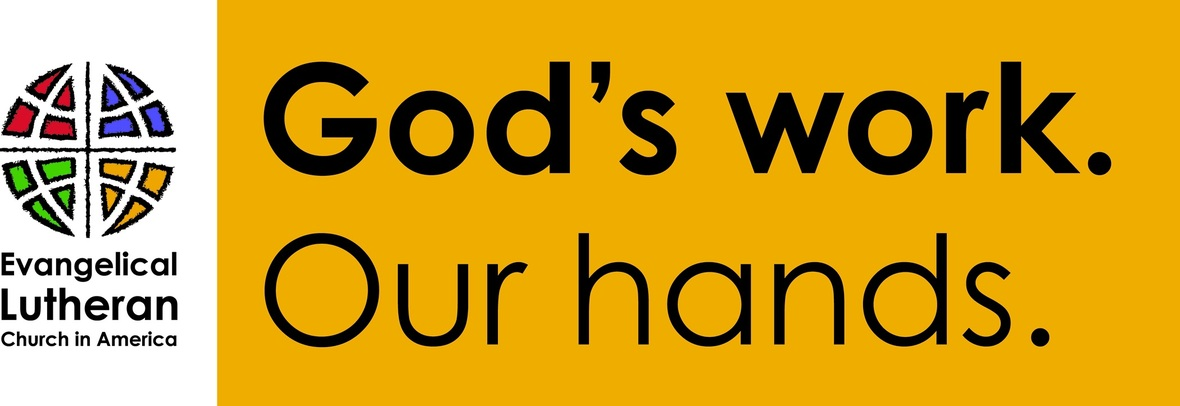/* styles */ God’s Work. Our Hands We will partner with the Northern Illinois Food Bank, St. Mark, and the youth ministry team to host a Mobile food pantry at St. Mark on three occasions this fall: Wednesday, Oct 21; Saturday, November 21; and Saturday, December 19. Volunteer commitment time would be from 10:30 A.M.-1:00 P.M. The food bank will bring food for 250 families. Everyone is welcome! We have our volunteers signed up for October. We still need volunteers for November and December. Volunteers need to arrive by 10:30 AM, wear a mask, bring work gloves if you have them, and dress for the weather. We will be done at 1:00 P.M. Please call or text 815-871-0390 or call the church office to sign up. You may also email melinda@stmarklc.com. Invite your friends, family, co-workers, teammates and classmates. This is a socially distanced event. We will be wearing masks and gloves! “Many hands make for light work.”
 table div table+table+table+table+table+table+table+table+table+table+table+table div table{width:100%;padding:0}table div table+table+table+table+table+table+table+table+table+table+table+table div table img{width:96.23%;padding:0;float:none}table div table+table+table+table+table+table+table+table+table+table+table+table div table td{width:100%;padding:0 1.88% 18px}/* styles */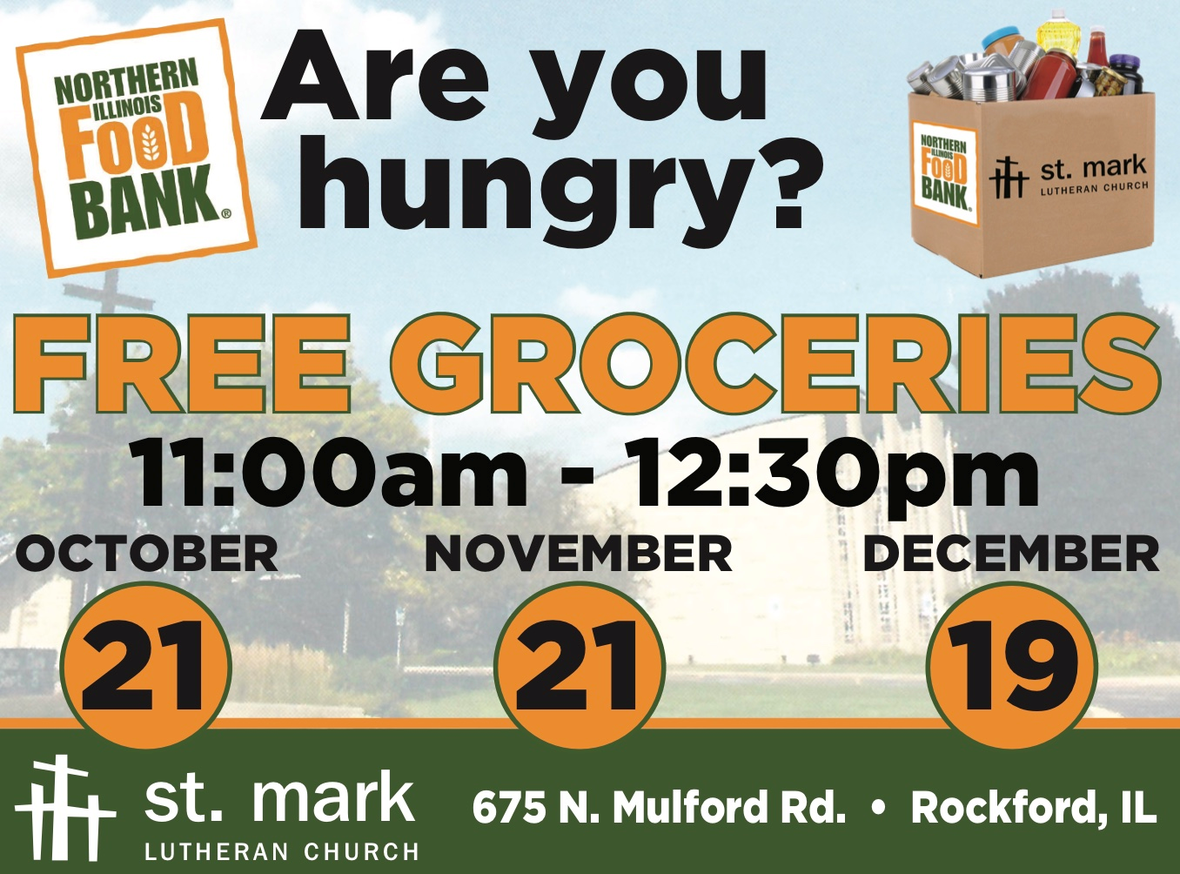table div table+table+table+table+table+table+table+table+table+table+table+table+table+table div table{width:100%;padding:0}table div table+table+table+table+table+table+table+table+table+table+table+table+table+table div table img{width:96.23%;padding:0;float:none}table div table+table+table+table+table+table+table+table+table+table+table+table+table+table div table td{width:100%;padding:0 1.88% 18px}/* styles */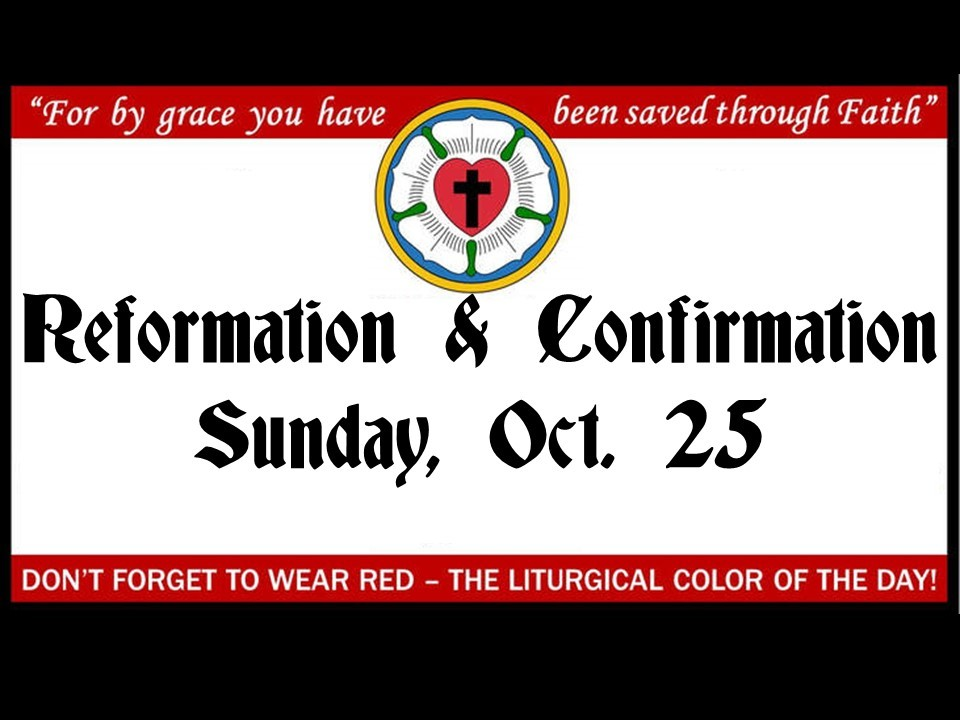Affirmation of Baptism
Next Sunday, October 25th, ninth-graders who have completed our two-year Confirmation Program will affirm their faith during worship. This is also Reformation Sunday, and everyone is encouraged to wear red in celebration of the Holy Spirit’s work in the lives of these confirmands, as well as all of God’s people.

 table div table+table+table+table+table+table+table+table+table+table+table+table+table+table+table+table div table{width:100%;padding:0}table div table+table+table+table+table+table+table+table+table+table+table+table+table+table+table+table div table img{width:96.23%;padding:0;float:none}table div table+table+table+table+table+table+table+table+table+table+table+table+table+table+table+table div table td{width:100%;padding:0 1.88% 18px}/* styles */Blood Drive
St. Mark will be sponsoring a blood drive on Tuesday, November 24 from 1:30 - 7:30 P.M. You can schedule a time by calling Jill Davenport at 815-398-3557 or by clicking on this link. The actual blood donation usually takes less than ten minutes. The entire process from the time you sign in to the time you leave takes about an hour. There are four steps to donating blood: medical history, mini-physical, donation and snacks. Please consider donating, the need is great!

 table div table+table+table+table+table+table+table+table+table+table+table+table+table+table+table+table+table+table div table{width:100%;padding:0}table div table+table+table+table+table+table+table+table+table+table+table+table+table+table+table+table+table+table div table img{width:96.23%;padding:0;float:none}table div table+table+table+table+table+table+table+table+table+table+table+table+table+table+table+table+table+table div table td{width:100%;padding:0 1.88% 18px}/* styles */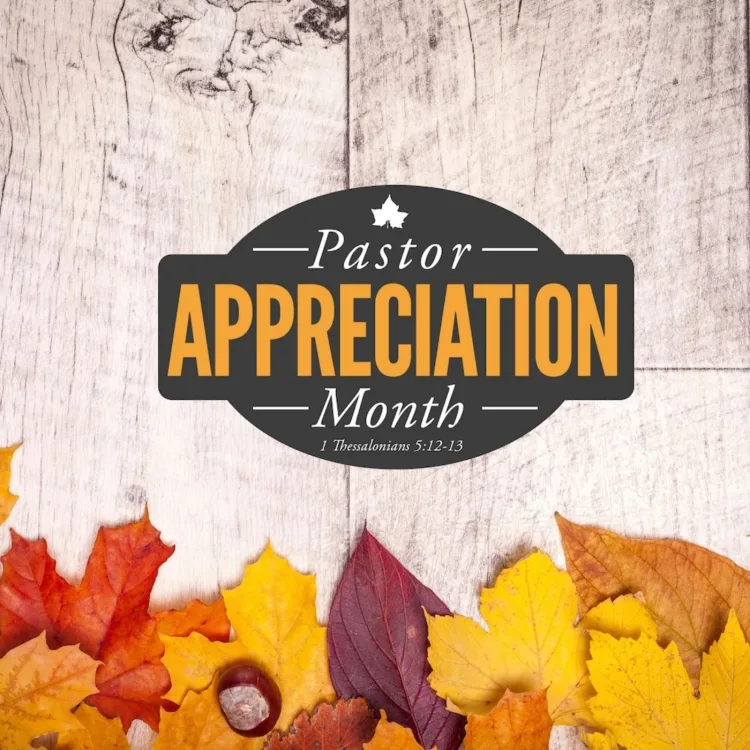October is Pastor Appreciation Month
Send our pastors; Chad, Robert, and Chuck a note or a card to let them know how much you appreciate their ministry to St. Mark. This has been a crazy year and they have provided guidance and direction to us all. Truly a blessing to St. Mark.

 table div table+table+table+table+table+table+table+table+table+table+table+table+table+table+table+table+table+table+table+table div table{width:100%;padding:0}table div table+table+table+table+table+table+table+table+table+table+table+table+table+table+table+table+table+table+table+table div table img{width:96.23%;padding:0;float:none}table div table+table+table+table+table+table+table+table+table+table+table+table+table+table+table+table+table+table+table+table div table td{width:100%;padding:0 1.88% 18px}/* styles */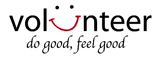Interested in helping out on Sunday mornings?
We are looking for someone to help during the 9:30 outdoor drive-in worship service with the sound. No prior experience necessary. Your job would be to run one microphone volume, iPad volume, master volume and play 2-3 tracks off the iPad. The band sets up their microphones. You would not have to commit to every Sunday. Please be at church by 9:00 am to get a quick tour of it all. Email Jill Davenport at jill@stmarklc.com or call the church office 815-398-3557. Thank you.

 table div table+table+table+table+table+table+table+table+table+table+table+table+table+table+table+table+table+table+table+table+table+table div table{width:100%;padding:0}table div table+table+table+table+table+table+table+table+table+table+table+table+table+table+table+table+table+table+table+table+table+table div table img{width:96.23%;padding:0;float:none}table div table+table+table+table+table+table+table+table+table+table+table+table+table+table+table+table+table+table+table+table+table+table div table td{width:100%;padding:0 1.88% 18px}/* styles */Volunteer Zoom Moderator Needed!
Sunday school is in need of a Zoom Moderator for our online classes! This is technical support - not teaching. This volunteer will admit families to the virtual classroom, help manage the group’s audio, watch the chat, and help with whatever other technical problems pop up along the way. I would love to have one dedicated volunteer who can help every Sunday, but if that’s not possible it can probably be a shared job between two people.

If you are a student who needs volunteer hours, this is a great way to earn them without leaving home!

Email julie@stmarklc.com with questions or to volunteer! Thank you!

 table div table+table+table+table+table+table+table+table+table+table+table+table+table+table+table+table+table+table+table+table+table+table+table+table div table{width:100%;padding:0}table div table+table+table+table+table+table+table+table+table+table+table+table+table+table+table+table+table+table+table+table+table+table+table+table div table img{width:96.23%;padding:0;float:none}table div table+table+table+table+table+table+table+table+table+table+table+table+table+table+table+table+table+table+table+table+table+table+table+table div table td{width:100%;padding:0 1.88% 18px}/* styles */St. Mark Mobile App!

This is different than our church directory app.
Our mobile app is designed specifically for St. Mark to improve our communication with our congregation and nurture our members. We encourage you to download the app. It's about getting involved and staying connected.

 table div table+table+table+table+table+table+table+table+table+table+table+table+table+table+table+table+table+table+table+table+table+table+table+table+table+table div table{width:100%;padding:0}table div table+table+table+table+table+table+table+table+table+table+table+table+table+table+table+table+table+table+table+table+table+table+table+table+table+table div table img{width:96.23%;padding:0;float:none}table div table+table+table+table+table+table+table+table+table+table+table+table+table+table+table+table+table+table+table+table+table+table+table+table+table+table div table td{width:100%;padding:0 1.88% 18px}/* styles */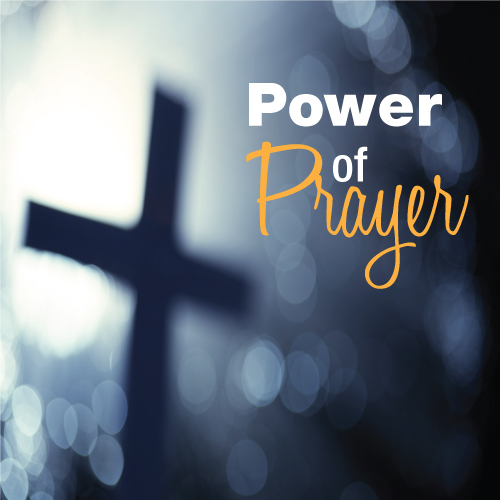St. Mark Prayer Chain
Would you like to be a part of the St. Mark prayer chain? Prayer requests come into the church office weekly. As we receive prayer requests we would send out an e-mail and ask that you pray daily for the persons mentioned in the requests. All requests are to remain confidential. Please e-mail melinda@stmarklc.com if you would like to participate in the prayer chain. “Be anxious for nothing, but in everything by prayer and supplication, with thanksgiving, let your requests be made known to God.” Colossians 4:2.

 table div table+table+table+table+table+table+table+table+table+table+table+table+table+table+table+table+table+table+table+table+table+table+table+table+table+table+table+table div table{width:100%;padding:0}table div table+table+table+table+table+table+table+table+table+table+table+table+table+table+table+table+table+table+table+table+table+table+table+table+table+table+table+table div table img{width:96.23%;padding:0;float:none}table div table+table+table+table+table+table+table+table+table+table+table+table+table+table+table+table+table+table+table+table+table+table+table+table+table+table+table+table div table td{width:100%;padding:0 1.88% 18px}/* styles */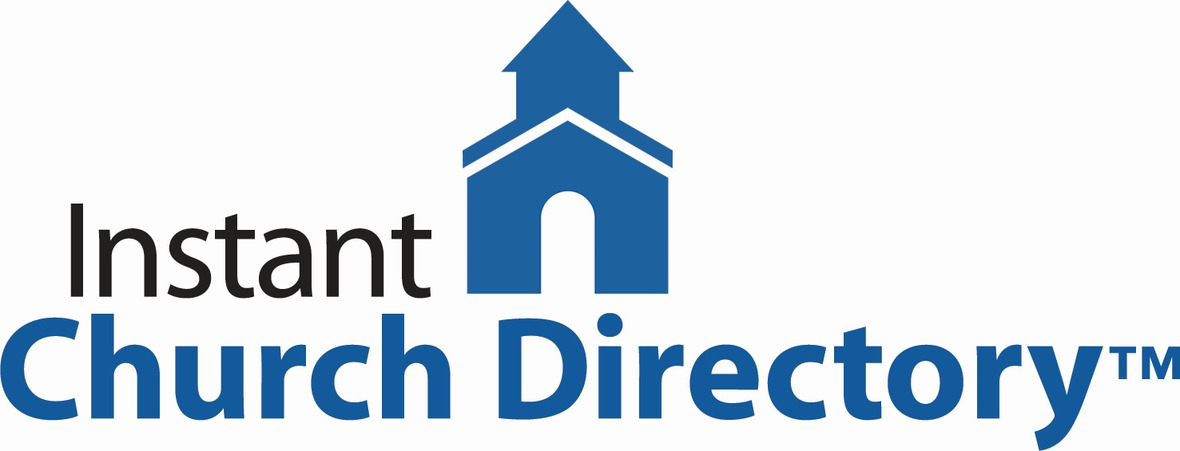Instant Church Directory
This is a great time to stay connected with our St. Mark family. If you need help downloading the app or creating an account on your computer or ipad, please call Jill Davenport at 815-218-3403 or email jill@stmarklc.com and she will be happy to help you through the process.

Instant Church Directory

Mobile App Login Instructions for Android & Kindle Devices

We also have a form for you to complete to make sure we have the most current information for the directory. There are paper forms at church or click on the link to Church Directory Form

 table div table+table+table+table+table+table+table+table+table+table+table+table+table+table+table+table+table+table+table+table+table+table+table+table+table+table+table+table+table+table div table{width:100%;padding:0}table div table+table+table+table+table+table+table+table+table+table+table+table+table+table+table+table+table+table+table+table+table+table+table+table+table+table+table+table+table+table div table img{width:96.23%;padding:0;float:none}table div table+table+table+table+table+table+table+table+table+table+table+table+table+table+table+table+table+table+table+table+table+table+table+table+table+table+table+table+table+table div table td{width:100%;padding:0 1.88% 18px}/* styles */Office Hours
Monday through Friday 9:00 A.M. - 1:00 P.M. As always, please call the office or Pastor Chad if you have any needs or concerns.

 table div table+table+table+table+table+table+table+table+table+table+table+table+table+table+table+table+table+table+table+table+table+table+table+table+table+table+table+table+table+table+table+table div table{width:100%;padding:0}table div table+table+table+table+table+table+table+table+table+table+table+table+table+table+table+table+table+table+table+table+table+table+table+table+table+table+table+table+table+table+table+table div table img{width:96.23%;padding:0;float:none}table div table+table+table+table+table+table+table+table+table+table+table+table+table+table+table+table+table+table+table+table+table+table+table+table+table+table+table+table+table+table+table+table div table td{width:100%;padding:0 1.88% 18px}/* styles */## Children's MinistryRemote Sunday School
Our online Sunday school classes have begun. If you haven't registered yet, please email Julie at julie@stmarklc.com.

We meet at 11:00 a.m. on Sundays. If the service runs a little long, we’ll adjust. See you on Sunday!

 table div table+table+table+table+table+table+table+table+table+table+table+table+table+table+table+table+table+table+table+table+table+table+table+table+table+table+table+table+table+table+table+table+table+table+table div table{width:100%;padding:0}table div table+table+table+table+table+table+table+table+table+table+table+table+table+table+table+table+table+table+table+table+table+table+table+table+table+table+table+table+table+table+table+table+table+table+table div table img{width:96.23%;padding:0;float:none}table div table+table+table+table+table+table+table+table+table+table+table+table+table+table+table+table+table+table+table+table+table+table+table+table+table+table+table+table+table+table+table+table+table+table+table div table td{width:100%;padding:0 1.88% 18px}/* styles */## Outreach Ministry

 table div table+table+table+table+table+table+table+table+table+table+table+table+table+table+table+table+table+table+table+table+table+table+table+table+table+table+table+table+table+table+table+table+table+table+table+table+table div table{width:100%;padding:0}table div table+table+table+table+table+table+table+table+table+table+table+table+table+table+table+table+table+table+table+table+table+table+table+table+table+table+table+table+table+table+table+table+table+table+table+table+table div table img{width:96.23%;padding:0;float:none}table div table+table+table+table+table+table+table+table+table+table+table+table+table+table+table+table+table+table+table+table+table+table+table+table+table+table+table+table+table+table+table+table+table+table+table+table+table div table td{width:100%;padding:0 1.88% 18px}/* styles */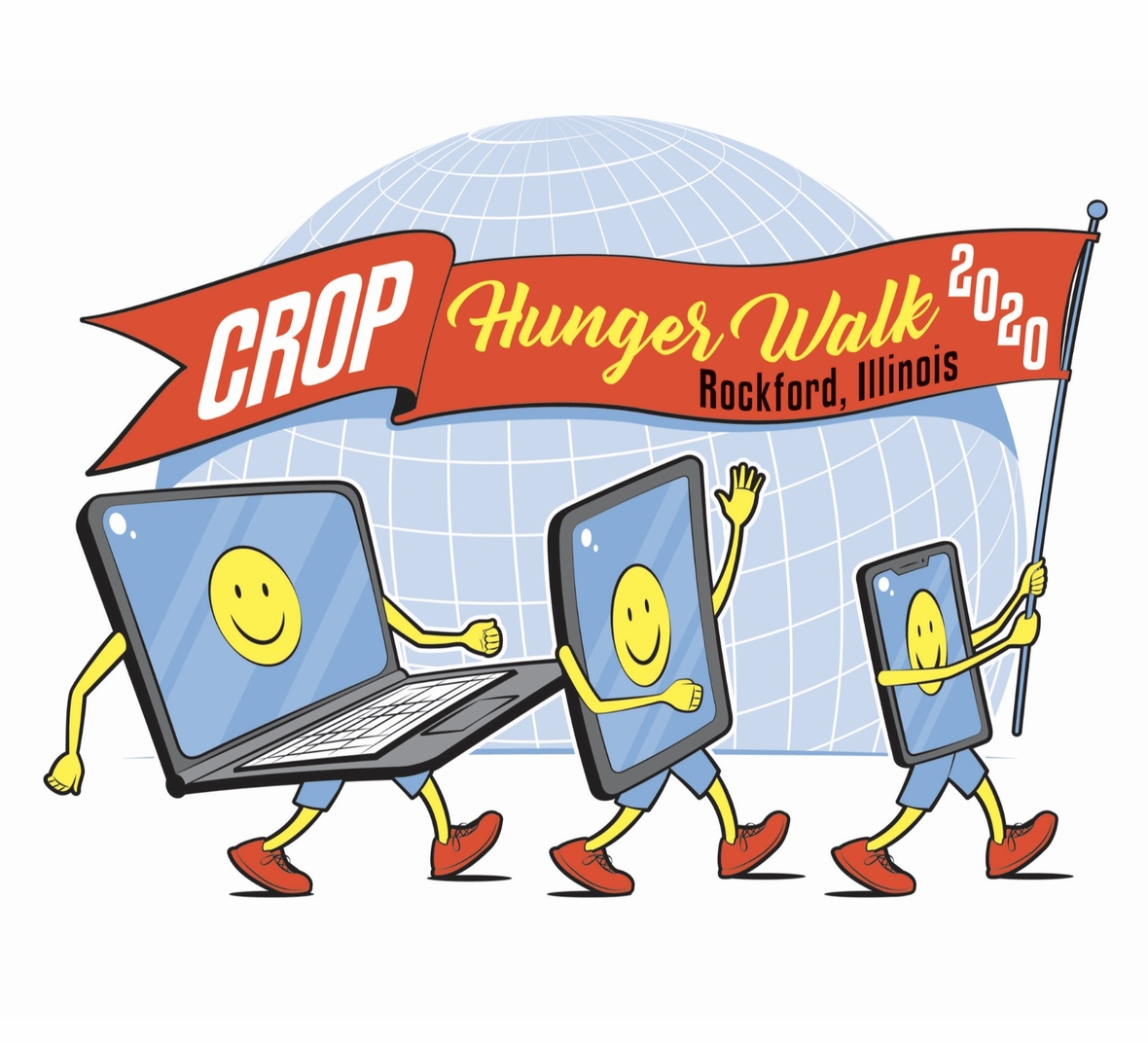Sunday is Crop Hunger Walk!

We are walking virtually. Please consider donating today. You may take out your phone, open your St. Mark “app,” look at the right bottom of the screen, click on the heart, scroll to Crop Hunger Walk 2020, and give! If you do not have the app, click here to go to our website. Give if you are able. Your generosity is appreciated!

 table div table+table+table+table+table+table+table+table+table+table+table+table+table+table+table+table+table+table+table+table+table+table+table+table+table+table+table+table+table+table+table+table+table+table+table+table+table+table+table div table{width:100%;padding:0}table div table+table+table+table+table+table+table+table+table+table+table+table+table+table+table+table+table+table+table+table+table+table+table+table+table+table+table+table+table+table+table+table+table+table+table+table+table+table+table div table img{width:96.23%;padding:0;float:none}table div table+table+table+table+table+table+table+table+table+table+table+table+table+table+table+table+table+table+table+table+table+table+table+table+table+table+table+table+table+table+table+table+table+table+table+table+table+table+table div table td{width:100%;padding:0 1.88% 18px}/* styles */Christmas Gifts for Children in Foster Care
The Outreach Committee is again sponsoring a drive to collect Christmas gifts for the over 500 area children who are in foster care in Northern Illinois.

Due to the 2020 pandemic health concerns, Lutheran Social Services is requesting gift cards for the kids instead of wrapped packages this year. We served 40 children last year and we are hoping to match or exceed this number as the need is so great.
The agency has requested gift cards, \$25 or \$30, from Target, Walmart, or any local shops where children would like to shop. We also will accept cash or check donations or donations marked for Christmas Gifts for Foster Care Children on the St. Mark App or through our website. Donations or gift cards can be dropped off or mailed to the church. The agency will purchase gift cards for the kids with the money that is donated.

The deadline for donations is November 30, so the agency will have time to get everything ready for the children’s Christmas. We hope our generous members will open their hearts for these children.

 table div table+table+table+table+table+table+table+table+table+table+table+table+table+table+table+table+table+table+table+table+table+table+table+table+table+table+table+table+table+table+table+table+table+table+table+table+table+table+table+table+table div table{width:100%;padding:0}table div table+table+table+table+table+table+table+table+table+table+table+table+table+table+table+table+table+table+table+table+table+table+table+table+table+table+table+table+table+table+table+table+table+table+table+table+table+table+table+table+table div table img{width:96.23%;padding:0;float:none}table div table+table+table+table+table+table+table+table+table+table+table+table+table+table+table+table+table+table+table+table+table+table+table+table+table+table+table+table+table+table+table+table+table+table+table+table+table+table+table+table+table div table td{width:100%;padding:0 1.88% 18px}/* styles */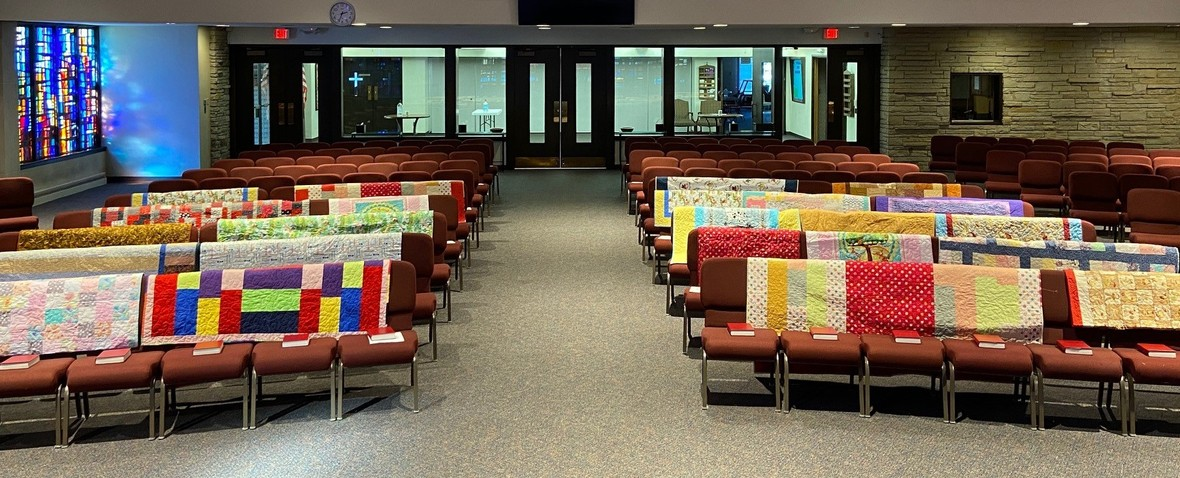/* styles */ Quilts for Habitat for Humanity Homes Our Sew and Sews have been very busy making quilts for each member of a Habitat home in Rockford. Many hours have been given to these beautiful quilts. Thank you ladies for thinking of others and making sure our families are taken care of. A warm hug from St. Mark.
 table div table+table+table+table+table+table+table+table+table+table+table+table+table+table+table+table+table+table+table+table+table+table+table+table+table+table+table+table+table+table+table+table+table+table+table+table+table+table+table+table+table+table+table+table div table{width:100%;padding:0}table div table+table+table+table+table+table+table+table+table+table+table+table+table+table+table+table+table+table+table+table+table+table+table+table+table+table+table+table+table+table+table+table+table+table+table+table+table+table+table+table+table+table+table+table div table img{width:96.23%;padding:0;float:none}table div table+table+table+table+table+table+table+table+table+table+table+table+table+table+table+table+table+table+table+table+table+table+table+table+table+table+table+table+table+table+table+table+table+table+table+table+table+table+table+table+table+table+table+table div table td{width:100%;padding:0 1.88% 18px}/* styles */## Choir rehearsal looks a little different this year!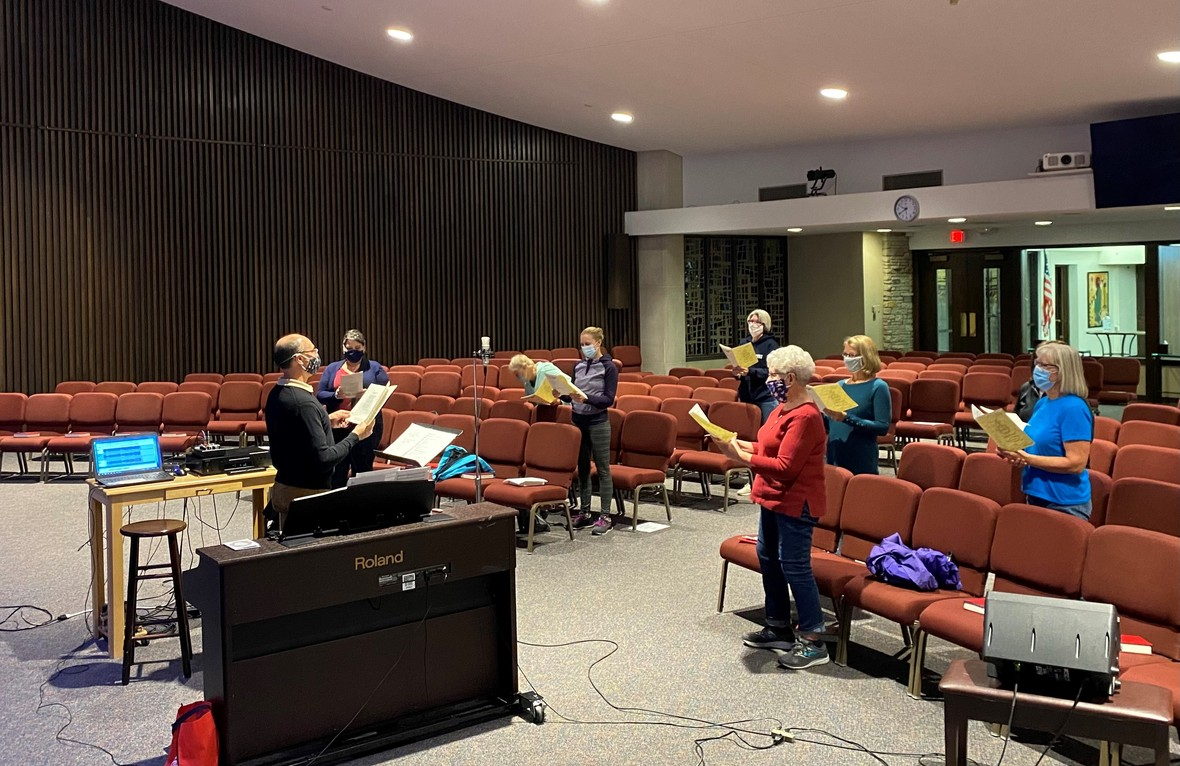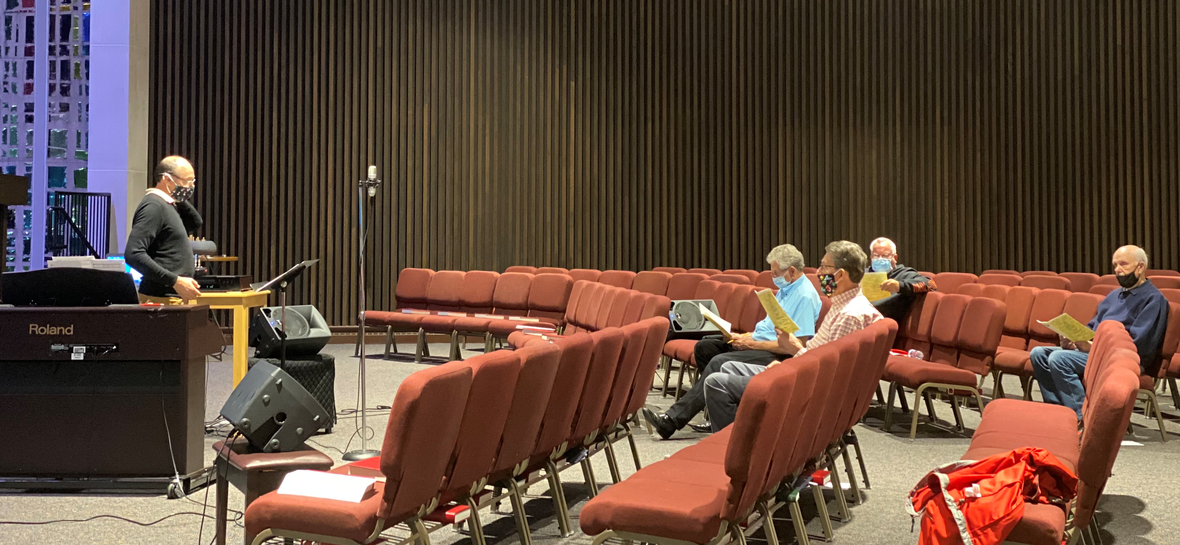table div table+table+table+table+table+table+table+table+table+table+table+table+table+table+table+table+table+table+table+table+table+table+table+table+table+table+table+table+table+table+table+table+table+table+table+table+table+table+table+table+table+table+table+table+table+table+table+table div table{width:100%;padding:0}table div table+table+table+table+table+table+table+table+table+table+table+table+table+table+table+table+table+table+table+table+table+table+table+table+table+table+table+table+table+table+table+table+table+table+table+table+table+table+table+table+table+table+table+table+table+table+table+table div table img{width:96.23%;padding:0;float:none}table div table+table+table+table+table+table+table+table+table+table+table+table+table+table+table+table+table+table+table+table+table+table+table+table+table+table+table+table+table+table+table+table+table+table+table+table+table+table+table+table+table+table+table+table+table+table+table+table div table td{width:100%;padding:0 1.88% 18px}/* styles */## Our St. Mark members:

Diane Swanson, Betty Adams, Nancy Scheel, Billie Reinhold, Jim Stewart, Constance Carlson, Marilyn Vivian, Sherri Lindquist, Tim Vivian, Bill Jennings, Tim Panjkovich, Bette Patterson, Jerry Cazaniga, David Young, Keith Haggestad.

## Family and friends of St. Mark members:

Butch Cotter, Wanda Adamson, Denise Nichols, Milane Bagel, Gayle Coffler, Darold Beekmann, Janet Webster, Rae Ann McIntire, Linda Johnson, Robert and Cindy Wilson, Pastor Jane McChesney, Emily Tropp, Louis Fivaz, Katelynn Audette, Eva Love Sherbondy, Norma Comstock, Carolyn King, Ashton Henley, Nathan Gyllin.

## Those serving in the military and their families:

Hunter Haggestad, Tristan Davenport, Scot Hornick, Bailey Panjkovich, Xander Gehrke, Jim Tammen, Brandon Ballenger, Taylor Eversole, Dean Barron.

Strengthen and encourage those in public health services and in the medical profession: care-givers, nurses, attendants, doctors, all who commit themselves to care for the sick and their families.

 table div table+table+table+table+table+table+table+table+table+table+table+table+table+table+table+table+table+table+table+table+table+table+table+table+table+table+table+table+table+table+table+table+table+table+table+table+table+table+table+table+table+table+table+table+table+table+table+table+table+table+table div table{width:100%;padding:0}table div table+table+table+table+table+table+table+table+table+table+table+table+table+table+table+table+table+table+table+table+table+table+table+table+table+table+table+table+table+table+table+table+table+table+table+table+table+table+table+table+table+table+table+table+table+table+table+table+table+table+table div table img{width:96.23%;padding:0;float:none}table div table+table+table+table+table+table+table+table+table+table+table+table+table+table+table+table+table+table+table+table+table+table+table+table+table+table+table+table+table+table+table+table+table+table+table+table+table+table+table+table+table+table+table+table+table+table+table+table+table+table+table div table td{width:100%;padding:0 1.88% 18px}/* styles *//* styles */ Office Hours Monday through Friday 9:00 A.M. - 1:00 P.M. Telephone: 815-398-3557 Publications St. Mark Memos, the weekly e-newsletter, is distributed each Thursday. The deadline for submission is Monday by 3:00 P.M. Please click to send submissions. Sermons Online Miss a Sunday at St. Mark? Please visit our website and watch the sermons online. Please click to watch.